### IMO Shortlist 1987 problem 1

Kvaliteta:
Avg: 0,0
Težina:
Avg: 0,0
Let f be a function that satisfies the following conditions: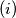$(i)$ If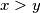$x > y$ and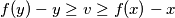$f(y) - y \geq v \geq f(x) - x$, then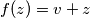$f(z) = v + z$, for some number$z$ between$x$ and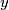$y$.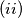$(ii)$ The equation$f(x) = 0$ has at least one solution, and among the solutions of this equation, there is one that is not smaller than all the other solutions;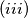$(iii)$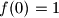$f(0) = 1$.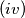$(iv)$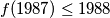$f(1987) \leq 1988$.$(v)$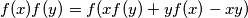$f(x)f(y) = f(xf(y) + yf(x) - xy)$.

Find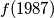$f(1987)$.

Proposed by Australia.
Izvor: Međunarodna matematička olimpijada, shortlist 1987